Next: About this document ... Up: lab_template Previous: lab_template

Subsections

# Assigning labels, expressions and functions

## Introduction

The purpose of this lab is to introduce you to the basics needed in any Maple lab. The main topics include: assigning labels, defining expressions, defining functions and plotting.

## Assigning labels

Suppose you want to assign a constant value to a variable name. Assigning labels is done by choosing a variable name followed by := and then the value to be assigned to that variable. For example, to let myname be assigned the value 1.5, you can type:
> myname := 1.5;

Once you hit return after this Maple line, any time the string of characters myname is used, it will have the value of 1.5. Note the use of the colon in front of the equal sign. This is necessary and if you forget the colon, Maple will not give an error message. It will appear as if you correctly assigned the value 1.5 to the label myname, however when you try to use myname again in the worksheet, it will not have the value 1.5.
Reusing variable names within the same lab is not recommended. It can be done, however, Maple will only store in its memory the last value that you assigned to the variable. So, if you go back to work on a previous problem with the same variable and forget to re-execute the old value, you could get some incorrect answers. Even if you do it correctly, sometimes Maple keeps a value in memory even though you've deleted the Maple line and tried to re-assign it. The best way to re-use a variable is to "clear" its memory for that variable. You can try the next example to see how this works.
> a := 5;
> a;
> a := 'a';
> a;

Once you have cleared the variable, you are free to use it again. Another thing to keep in mind is that if you close your Maple worksheet for any reason and reopen it, none of the commands will be stored in memory until you re-execute each command by hitting return after each of the Maple lines. This can also be done by clicking on the Edit menu and choosing Execute worksheet, however, this could be time consuming if there are a lot of plots in the worksheet.

## Entering an expression

Expressions such as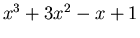can be entered in a similar way to variable assignment. Choose a variable name to represent the expression and assign the expression to the variable as follows.
> expr1 := x^3+3*x^2-x+1;

Note that in the expression above, there is an asterisk between 3 and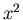. A common mistake is to write two functions next to each other without the "*" symbol. This would give incorrect results when using this expression since Maple doesn't understand implied multiplication. Another thing to keep in mind is when entering expressions in Maple that require the use of parentheses for grouping, the symbols ( and )'' should be used since square brackets and curley brackets have special purposes in Maple.

## Entering a function

Suppose you want to enter the same expression stored in expr1 above, but as a function of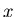. In Maple, you would type
> f := x-> x^3+3*x^2-x+1;

Below is how NOT'' to enter a function:
> f(x) := x^3+3*x^2-x+1;

The difference between expressions and functions are first the obvious, that expressions do not have to satisfy the definition of a function in the sense that for each input, there is a unique value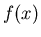. A function may be defined as an expression, but not all expressions can be defined as functions. The differences in Maple are numerous as you will see below when we evaluate the expression or function for a given value as well as when using the plot command.

## Evaluating functions and expressions

In order to evaluate an expression at a given value of, you must use the subs command. For example, if we wanted to evaluate the expression expr1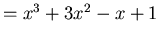atand at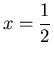, the examples below show how this can be done
> subs(x=2,expr1);
> subs(x=2.,expr1);
> subs(x=1/2,expr1);
> subs(x=0.5,expr1);

In the subs command, the first argument tells Maple what you would like to substitute in for. The second argument tells Maple what expression you are substituting into. Note the difference in outputs when a whole number or fraction is entered compared to a decimal. Here are a few more examples of evaluating expressions.
> subs(x=a+h,expr1);
> subs(theta=Pi,sin(theta)*cos(theta));

In Maple, functions are much easier to evaluate than expressions. In order to evaluate the function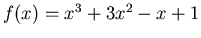at, then simply type
> f(2);

Here are a few more examples of evaluating functions.
> f(a+h);
> f(Pi);
> evalf(f(Pi));

Note the use of the evalf command in the last example above. This command is used when we want Maple to output the answer in decimal form. If this command is not used, the output to your Maple commands will be calculated analytically, where as the evalf command forces Maple to calculate the answers numerically. The evalf command has one essential argument, however a second argument can be added in order to tell how many digits we want to be in the answer. The example below evaluates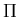using 10 digits, not 10 decimal places.
> evalf(Pi,10);


## Plotting

The following example shows how the Maple plot command is used to plot a function of one variable.
> f := x-> x^2;
> plot(f(x),x=-2..2);

The plot command above has two essential arguments. The first argument is the function(s) or expression(s) that you want to plot. The function or expression can be typed in before the plot command or you can simply type it in as the first argument. The second argument is the range of numbers for the-axis. The plot command allows you to add additional optional arguments as well. For instance, you may want to also restrict the y-axis range or add a title to your plot.
> plot(x^2,x=-2..2,y=-5..5,title=My First Plot);

This particular command allows you to add arguments, but if you were to leave off one of the essential arguments, you will get an error message. You can also plot more than one function or expression on the same graph by enclosing them in curly braces {}'' and separating them by commas. For example, we can plot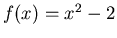and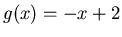on the same graph.
> f := x-> x^2-2;
> g := x-> -x+2;
> plot({f(x),g(x)},x=-4..4);


## Exercises

1. Enter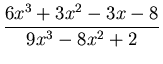as an expression.
1. Evaluate this expression atand at.
2. Evaluate this expression at.
3. Evaluate this expression at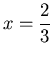and make sure your output is in decimal form.
4. Plot this expression over therange.
2. Repeat exercise 1 by first definingas a function.
3. Define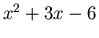and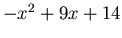as functions. Plot them both on the same graph. Estimate the two intersection points by observing the plot. Plug eachvalue back into both functions to show that the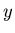values are the same.Next: About this document ... Up: lab_template Previous: lab_template
Dina Solitro
2004-08-24